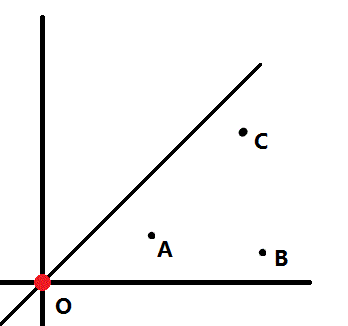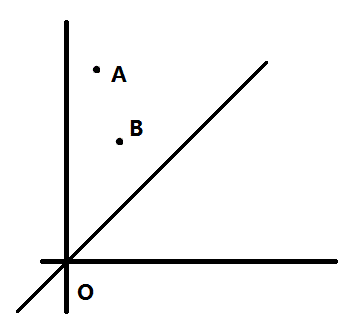# 曼哈顿距离

### 我们通常所指的距离是欧拉距离，这种距离体系很好的满足了三角形不等式，也合理地体现了空间中距离的大小关系。但是这一类距离在某些场合，甚至实际生活中却不太适用。1. 欧拉距离在进行了坐标系的线性变换之后依然不变，但是曼哈顿距离却会随坐标轴的方向变化。几种情况例外，就是当反转 x 轴或 y 轴，以及交换 x,y 轴时。
2. 到某个点曼哈顿距离相同的点的集合是一个斜的正方形。这个结论在思考和分析过程中非常有用。

# 曼哈顿距离生成树

### 如何求出曼哈顿距离生成树呢？### 引理 1：对于一个点，我们只需要连接每个 1/8 象限内的离它最近的点。

$$(O,A)+(A,B)=A_x+A_y+|B_x-A_x|+|B_y-A_y|$$
$$(O,A)+(O,B)=A_x+A_y+B_x+B_y$$

$$|B_x-A_x|+|B_y-A_y|<B_x+B_y$$

$$-A_x-A_y<0$$
$$A_y-A_x<2B_y$$
$$A_x-A_y<2B_x$$
$$A_x+A_y<2B_x+2B_y$$

### 寻找最近点$$A_y-A_x>=0$$
$$X>=0$$

### 扩展：

1. 如果点的范围比较大，需要进行离散化。
2. 对于动态更新的类似问题，请参考 http://www.doc88.com/p-998527952232.html

# 题目

#include <algorithm>
#include <iostream>
#include <cstring>
#include <cstdio>
#include <cmath>

#define MX 50005
#define MT 2000010
#define ME 1000005
#define MOV 1000001
#define oo 90000000
#define ls (node<<1)
#define rs (node<<1|1)
#define mid ((l+r)>>1)
#define dis(a,b) (abs((a).x-(b).x)+abs((a).y-(b).y))

using namespace std;

typedef struct point
{
int x,y,id;
}points;

typedef struct tnode
{
int mn,id;
}segtre;

points pot[MX];
segtre tre[MT<<2];
int fst[MX],nxt[ME],u[ME],v[ME],w[ME],lnum;
int fa[MX];
int ord[ME];
int n;

inline void addeg(int nu,int nv,int nw)
{
nxt[++lnum]=fst[nu];
fst[nu]=lnum;
u[lnum]=nu;
v[lnum]=nv;
w[lnum]=nw;
}

int findfa(int x)
{
return x==fa[x]?x:fa[x]=findfa(fa[x]);
}

inline void upd(int node)
{
if(tre[ls].mn<tre[rs].mn)tre[node].mn=tre[ls].mn,tre[node].id=tre[ls].id;
else tre[node].mn=tre[rs].mn,tre[node].id=tre[rs].id;
}

void build(int node,int l,int r)
{
tre[node].mn=+oo;
tre[node].id=-1;
if(l!=r)build(ls,l,mid),build(rs,mid+1,r);
}

void cng(int l,int r,int node,int p,int x,int id)
{
if(l==r)tre[node].mn=x,tre[node].id=id;
else if(p<=mid)cng(l,mid,ls,p,x,id),upd(node);
else cng(mid+1,r,rs,p,x,id),upd(node);
}

segtre qmn(int l,int r,int node,int ql,int qr)
{
segtre nul,a,b;
nul.mn=+oo;
nul.id=-1;
if(ql<=l&&r<=qr)return tre[node];
else if(ql>r||qr<l)return nul;
else
{
a=qmn(l,mid,ls,ql,qr);
b=qmn(mid+1,r,rs,ql,qr);
if(a.mn<b.mn)return a;
else return b;
}
}

inline bool cmpx(points a,points b)
{
return (a.x!=b.x)?(a.x>b.x):a.y>b.y;
}

inline bool cmpe(int a,int b)
{
return w[a]<w[b];
}

inline void sector1()
{
segtre near;
for(int i=1;i<=n;i++)
{
near=qmn(1,MOV<<1,1,MOV+pot[i].y-pot[i].x,+oo);
if(near.mn==+oo||near.id==-1);
else
{
}
cng(1,MOV<<1,1,MOV+pot[i].y-pot[i].x,pot[i].x+pot[i].y,pot[i].id);
}
}

long long cruskal()
{
long long ans=0,cnt=0;
for(int i=1;i<=n;i++)fa[i]=i;
for(int i=1;i<=lnum;i++)ord[i]=i;
sort(ord+1,ord+lnum+1,cmpe);
for(int i=1;i<=lnum;i++)
{
if(findfa(u[ord[i]])!=findfa(v[ord[i]]))
{
ans+=(long long)w[ord[i]];
cnt++;
fa[findfa(u[ord[i]])]=findfa(v[ord[i]]);
}
if(cnt>=n-1)break;
}
return ans;
}

void work()
{
sort(pot+1,pot+n+1,cmpx);
build(1,1,MOV<<1);
sector1();

for(int i=1;i<=n;i++)pot[i].x=-pot[i].x+MOV;
sort(pot+1,pot+n+1,cmpx);
build(1,1,MOV<<1);
sector1();

for(int i=1;i<=n;i++)swap(pot[i].x,pot[i].y);
sort(pot+1,pot+n+1,cmpx);
build(1,1,MOV<<1);
sector1();

for(int i=1;i<=n;i++)pot[i].y-=MOV,pot[i].y*=-1;
sort(pot+1,pot+n+1,cmpx);
build(1,1,MOV<<1);
sector1();

printf("%lld\n",cruskal());
}

void input()
{
scanf("%d",&n);
for(int i=1;i<=n;i++)scanf("%d%d",&pot[i].x,&pot[i].y),pot[i].id=i;
}

int main()
{
input();
work();
return 0;
}